Theory and Modern Applications

# Stability of an AQCQ-functional equation in paranormed spaces

## Abstract

Using the fixed point method and direct method, we prove the Hyers-Ulam stability of an additive-quadratic-cubic-quartic functional equation in paranormed spaces.

MSC:35A17, 39B52, 47H10, 39B72.

## 1 Introduction and preliminaries

The concept of statistical convergence for sequences of real numbers was introduced by Fast  and Steinhaus  independently, and since then several generalizations and applications of this notion have been investigated by various authors (see ). This notion was defined in normed spaces by Kolk .

We recall some basic facts concerning Fréchet spaces.

Definition 1.1 

Let X be a vector space. A paranorm $P:X\to \left[0,\mathrm{\infty }\right)$ is a function on X such that

1. (1)

$P\left(0\right)=0$;

2. (2)

$P\left(-x\right)=P\left(x\right)$;

3. (3)

$P\left(x+y\right)\le P\left(x\right)+P\left(y\right)$ (triangle inequality);

4. (4)

If $\left\{{t}_{n}\right\}$ is a sequence of scalars with ${t}_{n}\to t$ and $\left\{{x}_{n}\right\}\subset X$ with $P\left({x}_{n}-x\right)\to 0$, then $P\left({t}_{n}{x}_{n}-tx\right)\to 0$ (continuity of multiplication).

The pair $\left(X,P\right)$ is called a paranormed space if P is a paranorm on X.

The paranorm is called total if, in addition, we have

5. (5)

$P\left(x\right)=0$ implies $x=0$.

A Fréchet space is a total and complete paranormed space.

The stability problem of functional equations originated from the question of Ulam  concerning the stability of group homomorphisms. Hyers  gave the first affirmative partial answer to the question of Ulam for Banach spaces. Hyers’ theorem was generalized by Aoki  for additive mappings and by Th.M. Rassias  for linear mappings by considering an unbounded Cauchy difference. A generalization of the Th.M. Rassias theorem was obtained by Găvruta  by replacing the unbounded Cauchy difference by a general control function in the spirit of Th.M. Rassias’ approach.

In 1990 during the 27th International Symposium on Functional Equations, Th.M. Rassias  asked the question whether such a theorem can also be proved for $p\ge 1$. In 1991, following the same approach as in Th.M. Rassias , Gajda  gave an affirmative solution to this question for $p>1$. It was shown by Gajda , as well as by Th.M. Rassias and Šemrl , that one cannot prove a Th.M. Rassias’ type theorem when $p=1$ (cf. the books of P. Czerwik , D.H. Hyers, G. Isac and Th.M. Rassias ).

The functional equation

$f\left(x+y\right)+f\left(x-y\right)=2f\left(x\right)+2f\left(y\right)$

is called a quadratic functional equation. In particular, every solution of the quadratic functional equation is said to be a quadratic mapping. A Hyers-Ulam stability problem for the quadratic functional equation was proved by Skof  for mappings $f:X\to Y$, where X is a normed space and Y is a Banach space. Cholewa  noticed that the theorem of Skof is still true if the relevant domain X is replaced by an Abelian group. Czerwik  proved the Hyers-Ulam stability of the quadratic functional equation. The stability problems of several functional equations have been extensively investigated by a number of authors and there are many interesting results concerning this problem (see ).

In , Jun and Kim considered the following cubic functional equation:

$f\left(2x+y\right)+f\left(2x-y\right)=2f\left(x+y\right)+2f\left(x-y\right)+12f\left(x\right).$
(1.1)

It is easy to show that the function $f\left(x\right)={x}^{3}$ satisfies the functional equation (1.1), which is called a cubic functional equation, and every solution of the cubic functional equation is said to be a cubic mapping.

In , Lee et al. considered the following quartic functional equation:

$f\left(2x+y\right)+f\left(2x-y\right)=4f\left(x+y\right)+4f\left(x-y\right)+24f\left(x\right)-6f\left(y\right).$
(1.2)

It is easy to show that the function $f\left(x\right)={x}^{4}$ satisfies the functional equation (1.2), which is called a quartic functional equation, and every solution of the quartic functional equation is said to be a quartic mapping.

Throughout this paper, assume that $\left(X,P\right)$ is a Fréchet space and that $\left(Y,\parallel \cdot \parallel \right)$ is a Banach space.

In this paper, we prove the Hyers-Ulam stability of the following additive-quadratic-cubic-quartic functional equation

$\begin{array}{rcl}f\left(x+2y\right)+f\left(x-2y\right)& =& 4f\left(x+y\right)+4f\left(x-y\right)-6f\left(x\right)+f\left(2y\right)\\ +f\left(-2y\right)-4f\left(y\right)-4f\left(-y\right)\end{array}$
(1.3)

in paranormed spaces by using the fixed point method and direct method.

One can easily show that an odd mapping $f:X\to Y$ satisfies (1.3) if and only if the odd mapping $f:X\to Y$ is an additive-cubic mapping, i.e.,

$f\left(x+2y\right)+f\left(x-2y\right)=4f\left(x+y\right)+4f\left(x-y\right)-6f\left(x\right).$

It was shown in , Lemma 2.2] that $g\left(x\right):=f\left(2x\right)-2f\left(x\right)$ and $h\left(x\right):=f\left(2x\right)-8f\left(x\right)$ are cubic and additive, respectively, and that $f\left(x\right)=\frac{1}{6}g\left(x\right)-\frac{1}{6}h\left(x\right)$.

One can easily show that an even mapping $f:X\to Y$ satisfies (1.3) if and only if the even mapping $f:X\to Y$ is a quadratic-quartic mapping, i.e.,

$f\left(x+2y\right)+f\left(x-2y\right)=4f\left(x+y\right)+4f\left(x-y\right)-6f\left(x\right)+2f\left(2y\right)-8f\left(y\right).$

It was shown in , Lemma 2.1] that $g\left(x\right):=f\left(2x\right)-4f\left(x\right)$ and $h\left(x\right):=f\left(2x\right)-16f\left(x\right)$ are quartic and quadratic, respectively, and that $f\left(x\right)=\frac{1}{12}g\left(x\right)-\frac{1}{12}h\left(x\right)$.

## 2 Hyers-Ulam stability of the functional equation (1.3): an odd mapping case

For a given mapping f, we define

$\begin{array}{rcl}Df\left(x,y\right):& =& f\left(x+2y\right)+f\left(x-2y\right)-4f\left(x+y\right)-4f\left(x-y\right)+6f\left(x\right)\\ -f\left(2y\right)-f\left(-2y\right)+4f\left(y\right)+4f\left(-y\right).\end{array}$

Using the fixed point method and direct method, we prove the Hyers-Ulam stability of the functional equation $Df\left(x,y\right)=0$ in paranormed spaces: an odd mapping case.

Let S be a set. A function $m:S×S\to \left[0,\mathrm{\infty }\right]$ is called a generalized metric on S if m satisfies

1. (1)

$m\left(x,y\right)=0$ if and only if $x=y$;

2. (2)

$m\left(x,y\right)=m\left(y,x\right)$ for all $x,y\in S$;

3. (3)

$m\left(x,z\right)\le m\left(x,y\right)+m\left(y,z\right)$ for all $x,y,z\in S$.

We recall a fundamental result in the fixed point theory.

Theorem 2.1 [34, 35]

Let $\left(S,m\right)$ be a complete generalized metric space and let $J:S\to S$ be a strictly contractive mapping with Lipschitz constant $\alpha <1$. Then for each given element $x\in S$, either

$m\left({J}^{n}x,{J}^{n+1}x\right)=\mathrm{\infty }$

for all nonnegative integers n or there exists a positive integer ${n}_{0}$ such that

1. (1)

$m\left({J}^{n}x,{J}^{n+1}x\right)<\mathrm{\infty }$, $\mathrm{\forall }n\ge {n}_{0}$;

2. (2)

the sequence $\left\{{J}^{n}x\right\}$ converges to a fixed point ${y}^{\ast }$ of J;

3. (3)

${y}^{\ast }$ is the unique fixed point of J in the set $W=\left\{y\in S\mid m\left({J}^{{n}_{0}}x,y\right)<\mathrm{\infty }\right\}$;

4. (4)

$m\left(y,{y}^{\ast }\right)\le \frac{1}{1-\alpha }m\left(y,Jy\right)$ for all $y\in W$.

In 1996, Isac and Th.M. Rassias  were the first to provide applications of stability theory of functional equations for the proof of new fixed point theorems with applications. By using fixed point methods, the stability problems of several functional equations have been extensively investigated by a number of authors (see ).

Note that $P\left(2x\right)\le 2P\left(x\right)$ for all $x\in Y$.

Theorem 2.2 Let $\phi :{X}^{2}\to \left[0,\mathrm{\infty }\right)$ be a function such that there exists an $\alpha <1$ with

$\phi \left(x,y\right)\le 2\alpha \phi \left(\frac{x}{2},\frac{y}{2}\right)$
(2.1)

for all $x,y\in X$. Let $f:X\to Y$ be an odd mapping such that

$\parallel Df\left(x,y\right)\parallel \le \phi \left(x,y\right)$
(2.2)

for all $x,y\in X$. Then there exists a unique additive mapping $A:X\to Y$ such that

$\parallel f\left(2x\right)-8f\left(x\right)-A\left(x\right)\parallel \le \frac{1}{1-\alpha }\left(2\phi \left(x,x\right)+\frac{1}{2}\phi \left(2x,x\right)\right)$
(2.3)

for all $x\in X$.

Proof Letting $x=y$ in (2.2), we get

$\parallel f\left(3y\right)-4f\left(2y\right)+5f\left(y\right)\parallel \le \phi \left(y,y\right)$
(2.4)

for all $y\in X$.

Replacing x by 2y in (2.2), we get

$\parallel f\left(4y\right)-4f\left(3y\right)+6f\left(2y\right)-4f\left(y\right)\parallel \le \phi \left(2y,y\right)$
(2.5)

for all $y\in X$.

By (2.4) and (2.5),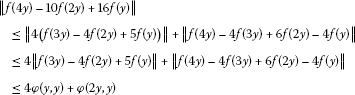(2.6)

for all $y\in X$. Replacing y by x and letting $g\left(x\right):=f\left(2x\right)-8f\left(x\right)$ in (2.6), we get

$\parallel g\left(x\right)-\frac{1}{2}g\left(2x\right)\parallel \le 2\phi \left(x,x\right)+\frac{1}{2}\phi \left(2x,x\right)$
(2.7)

for all $x\in X$.

Consider the set

$S:=\left\{h:X\to Y\right\}$

and introduce the generalized metric on S:

$m\left(k,h\right)=inf\left\{\mu \in {\mathbb{R}}_{+}:\parallel k\left(x\right)-h\left(x\right)\parallel \le \mu \left(2\phi \left(x,x\right)+\frac{1}{2}\phi \left(2x,x\right)\right),\mathrm{\forall }x\in X\right\},$

where, as usual, $inf\varphi =+\mathrm{\infty }$. It is easy to show that $\left(S,m\right)$ is complete (see , Lemma 2.1]).

Now we consider the linear mapping $J:S\to S$ such that

$Jh\left(x\right):=\frac{1}{2}h\left(2x\right)$

for all $x\in X$.

Let $k,h\in S$ be given such that $m\left(k,h\right)=\epsilon$. Since

$\parallel Jk\left(x\right)-Jh\left(x\right)\parallel =\parallel \frac{1}{2}k\left(2x\right)-\frac{1}{2}h\left(2x\right)\parallel \le \alpha \left(2\phi \left(x,x\right)+\frac{1}{2}\phi \left(2x,x\right)\right)$

for all $x\in X$, $m\left(k,h\right)=\epsilon$ implies that $m\left(Jk,Jh\right)\le \alpha \epsilon$. This means that

$m\left(Jk,Jh\right)\le \alpha m\left(k,h\right)$

for all $k,h\in S$.

It follows from (2.7) that $m\left(g,Jg\right)\le 1$.

By Theorem 2.1, there exists a mapping $A:X\to Y$ satisfying the following:

1. (1)

A is a fixed point of J, i.e.,

$A\left(2x\right)=2A\left(x\right)$
(2.8)

for all $x\in X$. The mapping A is a unique fixed point of J in the set

$M=\left\{k\in S:m\left(h,k\right)<\mathrm{\infty }\right\}.$

This implies that A is a unique mapping satisfying (2.8) such that there exists a $\mu \in \left(0,\mathrm{\infty }\right)$ satisfying

$\parallel g\left(x\right)-A\left(x\right)\parallel \le \mu \left(2\phi \left(x,x\right)+\frac{1}{2}\phi \left(2x,x\right)\right)$

for all $x\in X$;

2. (2)

$m\left({J}^{n}g,A\right)\to 0$ as $n\to \mathrm{\infty }$. This implies the equality

$\underset{n\to \mathrm{\infty }}{lim}\frac{1}{{2}^{n}}g\left({2}^{n}x\right)=A\left(x\right)$

for all $x\in X$;

3. (3)

$m\left(g,A\right)\le \frac{1}{1-\alpha }m\left(g,Jg\right)$, which implies the inequality

$m\left(g,A\right)\le \frac{1}{1-\alpha }.$

This implies that the inequality (2.3) holds true.

It follows from (2.1) and (2.2) that

$\begin{array}{rcl}\parallel DA\left(x,y\right)\parallel & =& \underset{n\to \mathrm{\infty }}{lim}\frac{1}{{2}^{n}}\parallel Dg\left({2}^{n}x,{2}^{n}y\right)\parallel \\ \le & \underset{n\to \mathrm{\infty }}{lim}\frac{1}{{2}^{n}}\left(\phi \left({2}^{n}\cdot 2x,{2}^{n}\cdot 2y\right)+8\phi \left({2}^{n}x,{2}^{n}y\right)\right)\\ \le & \underset{n\to \mathrm{\infty }}{lim}\left(\frac{{2}^{n}{\alpha }^{n}}{{2}^{n}}\phi \left(2x,2y\right)+8\frac{{2}^{n}{\alpha }^{n}}{{2}^{n}}\phi \left(x,y\right)\right)=0\end{array}$

for all $x,y\in X$. So $DA\left(x,y\right)=0$ for all $x,y\in X$. By , Lemma 2.2], $A:X\to Y$ is an additive mapping, as desired. □

Corollary 2.3 Let r be a positive real number with $r<1$, and let $f:X\to Y$ be an odd mapping such that

$\parallel Df\left(x,y\right)\parallel \le P{\left(x\right)}^{r}+P{\left(y\right)}^{r}$
(2.9)

for all $x,y\in X$. Then there exists a unique additive mapping $A:X\to Y$ such that

$\parallel f\left(2x\right)-8f\left(x\right)-A\left(x\right)\parallel \le \frac{9+{2}^{r}}{2-{2}^{r}}P{\left(x\right)}^{r}$
(2.10)

for all $x\in X$.

Proof Taking $\phi \left(x,y\right)=P{\left(x\right)}^{r}+P{\left(y\right)}^{r}$ for all $x,y\in X$ and choosing $\alpha ={2}^{r-1}$ in Theorem 2.2, we get the desired result. □

Theorem 2.4 Let $\phi :{X}^{2}\to \left[0,\mathrm{\infty }\right)$ be a function such that

$\mathrm{\Phi }\left(x,y\right):=\sum _{j=0}^{\mathrm{\infty }}\frac{1}{{2}^{j}}\phi \left({2}^{j}x,{2}^{j}y\right)<\mathrm{\infty }$

for all $x,y\in X$. Let $f:X\to Y$ be an odd mapping satisfying (2.2). Then there exists a unique additive mapping $A:X\to Y$ such that

$\parallel f\left(2x\right)-8f\left(x\right)-A\left(x\right)\parallel \le 2\mathrm{\Phi }\left(x,x\right)+\frac{1}{2}\mathrm{\Phi }\left(2x,x\right)$

for all $x\in X$.

Proof The proof is similar to the proof of , Theorem 2.2]. □

Remark 2.5 Let $r<1$. Letting $\phi \left(x,y\right)=P{\left(x\right)}^{r}+P{\left(y\right)}^{r}$ for all $x,y\in X$ in Theorem 2.4, we obtain the inequality (2.10). The proof is given in , Theorem 2.2].

Theorem 2.6 Let $\phi :{Y}^{2}\to \left[0,\mathrm{\infty }\right)$ be a function such that there exists an $\alpha <1$ with

$\phi \left(x,y\right)\le \frac{\alpha }{2}\phi \left(2x,2y\right)$
(2.11)

for all $x,y\in Y$. Let $f:Y\to X$ be an odd mapping such that

$P\left(Df\left(x,y\right)\right)\le \phi \left(x,y\right)$
(2.12)

for all $x,y\in Y$. Then there exists a unique additive mapping $A:Y\to X$ such that

$P\left(f\left(2x\right)-8f\left(x\right)-A\left(x\right)\right)\le \frac{\alpha }{1-\alpha }\left(2\phi \left(x,x\right)+\frac{1}{2}\phi \left(2x,x\right)\right)$
(2.13)

for all $x\in Y$.

Proof Letting $x=y$ in (2.12), we get

$P\left(f\left(3y\right)-4f\left(2y\right)+5f\left(y\right)\right)\le \phi \left(y,y\right)$
(2.14)

for all $y\in Y$.

Replacing x by 2y in (2.12), we get

$P\left(f\left(4y\right)-4f\left(3y\right)+6f\left(2y\right)-4f\left(y\right)\right)\le \phi \left(2y,y\right)$
(2.15)

for all $y\in Y$.

By (2.14) and (2.15),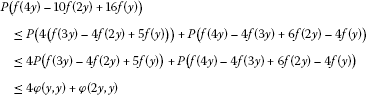(2.16)

for all $y\in X$. Replacing y by $\frac{x}{2}$ and letting $g\left(x\right):=f\left(2x\right)-8f\left(x\right)$ in (2.16), we get

$P\left(g\left(x\right)-2g\left(\frac{x}{2}\right)\right)\le 4\phi \left(\frac{x}{2},\frac{x}{2}\right)+\phi \left(x,\frac{x}{2}\right)\le \alpha \left(2\phi \left(x,x\right)+\frac{1}{2}\phi \left(2x,x\right)\right)$
(2.17)

for all $x\in Y$.

Consider the set

$S:=\left\{h:Y\to X\right\}$

and introduce the generalized metric on S:

$m\left(k,h\right)=inf\left\{\mu \in {\mathbb{R}}_{+}:P\left(k\left(x\right)-h\left(x\right)\right)\le \mu \left(2\phi \left(x,x\right)+\frac{1}{2}\phi \left(2x,x\right)\right),\mathrm{\forall }x\in Y\right\},$

where, as usual, $inf\varphi =+\mathrm{\infty }$. It is easy to show that $\left(S,m\right)$ is complete (see , Lemma 2.1]).

Now we consider the linear mapping $J:S\to S$ such that

$Jh\left(x\right):=2h\left(\frac{x}{2}\right)$

for all $x\in Y$.

Let $k,h\in S$ be given such that $m\left(k,h\right)=\epsilon$. Since

$P\left(Jk\left(x\right)-Jh\left(x\right)\right)=P\left(2k\left(\frac{x}{2}\right)-2h\left(\frac{x}{2}\right)\right)\le \alpha \left(2\phi \left(x,x\right)+\frac{1}{2}\phi \left(2x,x\right)\right)$

for all $x\in Y$, $m\left(k,h\right)=\epsilon$ implies that $m\left(Jk,Jh\right)\le \alpha \epsilon$. This means that

$m\left(Jk,Jh\right)\le \alpha m\left(k,h\right)$

for all $k,h\in S$.

It follows from (2.17) that $m\left(g,Jg\right)\le \alpha$.

By Theorem 2.1, there exists a mapping $A:X\to Y$ satisfying the following:

1. (1)

A is a fixed point of J, i.e.,

$A\left(\frac{x}{2}\right)=\frac{1}{2}A\left(x\right)$
(2.18)

for all $x\in X$. The mapping A is a unique fixed point of J in the set

$M=\left\{k\in S:m\left(h,k\right)<\mathrm{\infty }\right\}.$

This implies that A is a unique mapping satisfying (2.18) such that there exists a $\mu \in \left(0,\mathrm{\infty }\right)$ satisfying

$P\left(g\left(x\right)-A\left(x\right)\right)\le \mu \left(2\phi \left(x,x\right)+\frac{1}{2}\phi \left(2x,x\right)\right)$

for all $x\in Y$;

2. (2)

$m\left({J}^{n}g,A\right)\to 0$ as $n\to \mathrm{\infty }$. This implies the equality

$\underset{n\to \mathrm{\infty }}{lim}{2}^{n}g\left(\frac{x}{{2}^{n}}\right)=A\left(x\right)$

for all $x\in Y$;

3. (3)

$m\left(g,A\right)\le \frac{1}{1-\alpha }m\left(g,Jg\right)$, which implies the inequality

$m\left(g,A\right)\le \frac{\alpha }{1-\alpha }.$

This implies that the inequality (2.13) holds true.

It follows from (2.11) and (2.12) that

$\begin{array}{rcl}P\left(DA\left(x,y\right)\right)& =& \underset{n\to \mathrm{\infty }}{lim}P\left({2}^{n}\left(Dg\left(\frac{x}{{2}^{n}},\frac{y}{{2}^{n}}\right)\right)\right)\\ \le & \underset{n\to \mathrm{\infty }}{lim}{2}^{n}P\left(Dg\left(\frac{x}{{2}^{n}},\frac{y}{{2}^{n}}\right)\right)\\ \le & \underset{n\to \mathrm{\infty }}{lim}{2}^{n}\left(\phi \left(\frac{2x}{{2}^{n}},\frac{2y}{{2}^{n}}\right)+8\phi \left(\frac{x}{{2}^{n}},\frac{y}{{2}^{n}}\right)\right)\\ \le & \underset{n\to \mathrm{\infty }}{lim}\left(\frac{{2}^{n}{\alpha }^{n}}{{2}^{n}}\phi \left(2x,2y\right)+8\frac{{2}^{n}{\alpha }^{n}}{{2}^{n}}\phi \left(x,y\right)\right)=0\end{array}$

for all $x,y\in Y$. So $DA\left(x,y\right)=0$ for all $x,y\in Y$. By , Lemma 2.2], $A:Y\to X$ is an additive mapping, as desired. □

Corollary 2.7 Let r, θ be positive real numbers with $r>1$, and let $f:Y\to X$ be an odd mapping such that

$P\left(Df\left(x,y\right)\right)\le \theta \left({\parallel x\parallel }^{r}+{\parallel y\parallel }^{r}\right)$
(2.19)

for all $x,y\in Y$. Then there exists a unique additive mapping $A:Y\to X$ such that

$P\left(f\left(2x\right)-8f\left(x\right)-A\left(x\right)\right)\le \frac{9+{2}^{r}}{{2}^{r}-2}\theta {\parallel x\parallel }^{r}$
(2.20)

for all $x\in Y$.

Proof Taking $\phi \left(x,y\right)=\theta \left({\parallel x\parallel }^{r}+{\parallel y\parallel }^{r}\right)$ for all $x,y\in X$ and choosing $\alpha ={2}^{1-r}$ in Theorem 2.6, we get the desired result. □

Theorem 2.8 Let $\phi :{Y}^{2}\to \left[0,\mathrm{\infty }\right)$ be a function such that

$\mathrm{\Phi }\left(x,y\right):=\sum _{j=1}^{\mathrm{\infty }}{2}^{j}\phi \left(\frac{x}{{2}^{j}},\frac{y}{{2}^{j}}\right)<\mathrm{\infty }$

for all $x,y\in Y$. Let $f:Y\to X$ be an odd mapping satisfying (2.12). Then there exists a unique additive mapping $A:Y\to X$ such that

$P\left(f\left(2x\right)-8f\left(x\right)-A\left(x\right)\right)\le 2\mathrm{\Phi }\left(x,x\right)+\frac{1}{2}\mathrm{\Phi }\left(2x,x\right)$

for all $x\in Y$.

Proof The proof is similar to the proof of , Theorem 2.1]. □

Remark 2.9 Let $r>1$. Letting $\phi \left(x,y\right)=\theta \left({\parallel x\parallel }^{r}+{\parallel y\parallel }^{r}\right)$ for all $x,y\in X$ in Theorem 2.8, we obtain the inequality (2.20). The proof is given in , Theorem 2.1].

Theorem 2.10 Let $\phi :{X}^{2}\to \left[0,\mathrm{\infty }\right)$ be a function such that there exists an $\alpha <1$ with

$\phi \left(x,y\right)\le 8\alpha \phi \left(\frac{x}{2},\frac{y}{2}\right)$
(2.21)

for all $x,y\in X$. Let $f:X\to Y$ be an odd mapping satisfying (2.2). Then there exists a unique cubic mapping $C:X\to Y$ such that

$\parallel f\left(2x\right)-2f\left(x\right)-C\left(x\right)\parallel \le \frac{1}{1-\alpha }\left(\frac{1}{2}\phi \left(x,x\right)+\frac{1}{8}\phi \left(2x,x\right)\right)$
(2.22)

for all $x\in X$.

Proof Replacing y by x and letting $g\left(x\right):=f\left(2x\right)-2f\left(x\right)$ in (2.6), we get

$\parallel g\left(x\right)-\frac{1}{8}g\left(2x\right)\parallel \le \frac{1}{2}\phi \left(x,x\right)+\frac{1}{8}\phi \left(2x,x\right)$
(2.23)

for all $x\in X$.

Consider the set

$S:=\left\{h:X\to Y\right\}$

and introduce the generalized metric on S:

$m\left(k,h\right)=inf\left\{\mu \in {\mathbb{R}}_{+}:\parallel k\left(x\right)-h\left(x\right)\parallel \le \mu \left(\frac{1}{2}\phi \left(x,x\right)+\frac{1}{8}\phi \left(2x,x\right)\right),\mathrm{\forall }x\in X\right\},$

where, as usual, $inf\varphi =+\mathrm{\infty }$. It is easy to show that $\left(S,m\right)$ is complete (see , Lemma 2.1]).

Now we consider the linear mapping $J:S\to S$ such that

$Jh\left(x\right):=\frac{1}{8}h\left(2x\right)$

for all $x\in X$.

Let $k,h\in S$ be given such that $m\left(k,h\right)=\epsilon$. Since

$\parallel Jk\left(x\right)-Jh\left(x\right)\parallel =\parallel \frac{1}{8}k\left(2x\right)-\frac{1}{8}h\left(2x\right)\parallel \le \alpha \left(\frac{1}{2}\phi \left(x,x\right)+\frac{1}{8}\phi \left(2x,x\right)\right)$

for all $x\in X$, $m\left(k,h\right)=\epsilon$ implies that $m\left(Jk,Jh\right)\le \alpha \epsilon$. This means that

$m\left(Jk,Jh\right)\le \alpha m\left(k,h\right)$

for all $k,h\in S$.

It follows from (2.23) that $m\left(g,Jg\right)\le 1$.

By Theorem 2.1, there exists a mapping $C:X\to Y$ satisfying the following:

1. (1)

C is a fixed point of J, i.e.,

$C\left(2x\right)=8C\left(x\right)$
(2.24)

for all $x\in X$. The mapping C is a unique fixed point of J in the set

$M=\left\{k\in S:m\left(h,k\right)<\mathrm{\infty }\right\}.$

This implies that C is a unique mapping satisfying (2.24) such that there exists a $\mu \in \left(0,\mathrm{\infty }\right)$ satisfying

$\parallel g\left(x\right)-C\left(x\right)\parallel \le \mu \left(\frac{1}{2}\phi \left(x,x\right)+\frac{1}{8}\phi \left(2x,x\right)\right)$

for all $x\in X$;

2. (2)

$m\left({J}^{n}g,C\right)\to 0$ as $n\to \mathrm{\infty }$. This implies the equality

$\underset{n\to \mathrm{\infty }}{lim}\frac{1}{{8}^{n}}g\left({2}^{n}x\right)=C\left(x\right)$

for all $x\in X$;

3. (3)

$m\left(g,C\right)\le \frac{1}{1-\alpha }m\left(g,Jg\right)$, which implies the inequality

$m\left(g,C\right)\le \frac{1}{1-\alpha }.$

This implies that the inequality (2.22) holds true.

It follows from (2.2) and (2.21) that

$\begin{array}{rcl}\parallel DC\left(x,y\right)\parallel & =& \underset{n\to \mathrm{\infty }}{lim}\frac{1}{{8}^{n}}\parallel Dg\left({2}^{n}x,{2}^{n}y\right)\parallel \\ \le & \underset{n\to \mathrm{\infty }}{lim}\frac{1}{{8}^{n}}\left(\phi \left({2}^{n}\cdot 2x,{2}^{n}\cdot 2y\right)+2\phi \left({2}^{n}x,{2}^{n}y\right)\right)\\ \le & \underset{n\to \mathrm{\infty }}{lim}\left(\frac{{8}^{n}{\alpha }^{n}}{{8}^{n}}\phi \left(2x,2y\right)+2\frac{{8}^{n}{\alpha }^{n}}{{8}^{n}}\phi \left(x,y\right)\right)=0\end{array}$

for all $x,y\in X$. So $DC\left(x,y\right)=0$ for all $x,y\in X$. By , Lemma 2.2], $C:X\to Y$ is a cubic mapping, as desired. □

Corollary 2.11 Let r be a positive real number with $r<3$, and let $f:X\to Y$ be an odd mapping satisfying (2.9). Then there exists a unique cubic mapping $C:X\to Y$ such that

$\parallel f\left(2x\right)-2f\left(x\right)-C\left(x\right)\parallel \le \frac{9+{2}^{r}}{8-{2}^{r}}P{\left(x\right)}^{r}$
(2.25)

for all $x\in X$.

Proof Taking $\phi \left(x,y\right)=P{\left(x\right)}^{r}+P{\left(y\right)}^{r}$ for all $x,y\in X$ and choosing $\alpha ={2}^{r-3}$ in Theorem 2.10, we get the desired result. □

Theorem 2.12 Let $\phi :{X}^{2}\to \left[0,\mathrm{\infty }\right)$ be a function such that

$\mathrm{\Phi }\left(x,y\right):=\sum _{j=0}^{\mathrm{\infty }}\frac{1}{{8}^{j}}\phi \left({2}^{j}x,{2}^{j}y\right)<\mathrm{\infty }$

for all $x,y\in X$. Let $f:X\to Y$ be an odd mapping satisfying (2.2). Then there exists a unique cubic mapping $C:X\to Y$ such that

$\parallel f\left(2x\right)-2f\left(x\right)-C\left(x\right)\parallel \le \frac{1}{2}\mathrm{\Phi }\left(x,x\right)+\frac{1}{8}\mathrm{\Phi }\left(2x,x\right)$

for all $x\in X$.

Proof The proof is similar to the proof of , Theorem 2.4]. □

Remark 2.13 Let $r<3$. Letting $\phi \left(x,y\right)=P{\left(x\right)}^{r}+P{\left(y\right)}^{r}$ for all $x,y\in X$ in Theorem 2.12, we obtain the inequality (2.25). The proof is given in , Theorem 2.4].

Theorem 2.14 Let $\phi :{Y}^{2}\to \left[0,\mathrm{\infty }\right)$ be a function such that there exists an $\alpha <1$ with

$\phi \left(x,y\right)\le \frac{\alpha }{8}\phi \left(2x,2y\right)$
(2.26)

for all $x,y\in Y$. Let $f:Y\to X$ be an odd mapping satisfying (2.12). Then there exists a unique cubic mapping $C:Y\to X$ such that

$P\left(f\left(2x\right)-2f\left(x\right)-C\left(x\right)\right)\le \frac{\alpha }{1-\alpha }\left(\frac{1}{2}\phi \left(x,x\right)+\frac{1}{8}\phi \left(2x,x\right)\right)$
(2.27)

for all $x\in Y$.

Proof Replacing y by $\frac{x}{2}$ and letting $g\left(x\right):=f\left(2x\right)-2f\left(x\right)$ in (2.16), we get

$P\left(g\left(x\right)-8g\left(\frac{x}{2}\right)\right)\le 4\phi \left(\frac{x}{2},\frac{x}{2}\right)+\phi \left(x,\frac{x}{2}\right)\le \alpha \left(\frac{1}{2}\phi \left(x,x\right)+\frac{1}{8}\phi \left(2x,x\right)\right)$
(2.28)

for all $x\in Y$.

Consider the set

$S:=\left\{h:Y\to X\right\}$

and introduce the generalized metric on S:

$m\left(k,h\right)=inf\left\{\mu \in {\mathbb{R}}_{+}:P\left(k\left(x\right)-h\left(x\right)\right)\le \mu \left(\frac{1}{2}\phi \left(x,x\right)+\frac{1}{8}\phi \left(2x,x\right)\right),\mathrm{\forall }x\in Y\right\},$

where, as usual, $inf\varphi =+\mathrm{\infty }$. It is easy to show that $\left(S,m\right)$ is complete (see , Lemma 2.1]).

Now we consider the linear mapping $J:S\to S$ such that

$Jh\left(x\right):=8h\left(\frac{x}{2}\right)$

for all $x\in Y$.

Let $k,h\in S$ be given such that $m\left(k,h\right)=\epsilon$. Since

$P\left(Jk\left(x\right)-Jh\left(x\right)\right)=P\left(8k\left(\frac{x}{2}\right)-8h\left(\frac{x}{2}\right)\right)\le \alpha \left(\frac{1}{2}\phi \left(x,x\right)+\frac{1}{8}\phi \left(2x,x\right)\right)$

for all $x\in Y$, $m\left(k,h\right)=\epsilon$ implies that $m\left(Jk,Jh\right)\le \alpha \epsilon$. This means that

$m\left(Jk,Jh\right)\le \alpha m\left(k,h\right)$

for all $k,h\in S$.

It follows from (2.28) that $m\left(g,Jg\right)\le \alpha$.

By Theorem 2.1, there exists a mapping $C:X\to Y$ satisfying the following:

1. (1)

C is a fixed point of J, i.e.,

$C\left(\frac{x}{2}\right)=\frac{1}{8}C\left(x\right)$
(2.29)

for all $x\in X$. The mapping C is a unique fixed point of J in the set

$M=\left\{k\in S:m\left(h,k\right)<\mathrm{\infty }\right\}.$

This implies that C is a unique mapping satisfying (2.29) such that there exists a $\mu \in \left(0,\mathrm{\infty }\right)$ satisfying

$P\left(g\left(x\right)-C\left(x\right)\right)\le \mu \left(\frac{1}{2}\phi \left(x,x\right)+\frac{1}{8}\phi \left(2x,x\right)\right)$

for all $x\in Y$;

2. (2)

$m\left({J}^{n}g,C\right)\to 0$ as $n\to \mathrm{\infty }$. This implies the equality

$\underset{n\to \mathrm{\infty }}{lim}{8}^{n}g\left(\frac{x}{{2}^{n}}\right)=C\left(x\right)$

for all $x\in Y$;

3. (3)

$m\left(g,C\right)\le \frac{1}{1-\alpha }m\left(g,Jg\right)$, which implies the inequality

$m\left(g,C\right)\le \frac{\alpha }{1-\alpha }.$

This implies that the inequality (2.27) holds true.

It follows from (2.12) and (2.26) that

$\begin{array}{rcl}P\left(DC\left(x,y\right)\right)& =& \underset{n\to \mathrm{\infty }}{lim}P\left({8}^{n}\left(Dg\left(\frac{x}{{2}^{n}},\frac{y}{{2}^{n}}\right)\right)\right)\\ \le & \underset{n\to \mathrm{\infty }}{lim}{8}^{n}P\left(Dg\left(\frac{x}{{2}^{n}},\frac{y}{{2}^{n}}\right)\right)\\ \le & \underset{n\to \mathrm{\infty }}{lim}{8}^{n}\left(\phi \left(\frac{2x}{{2}^{n}},\frac{2y}{{2}^{n}}\right)+2\phi \left(\frac{x}{{2}^{n}},\frac{y}{{2}^{n}}\right)\right)\\ \le & \underset{n\to \mathrm{\infty }}{lim}\left(\frac{{8}^{n}{\alpha }^{n}}{{8}^{n}}\phi \left(2x,2y\right)+2\frac{{8}^{n}{\alpha }^{n}}{{8}^{n}}\phi \left(x,y\right)\right)=0\end{array}$

for all $x,y\in Y$. So $DC\left(x,y\right)=0$ for all $x,y\in Y$. By , Lemma 2.2], $C:Y\to X$ is a cubic mapping, as desired. □

Corollary 2.15 Let r, θ be positive real numbers with $r>3$, and let $f:Y\to X$ be an odd mapping satisfying (2.19). Then there exists a unique cubic mapping $C:Y\to X$ such that

$P\left(f\left(2x\right)-2f\left(x\right)-C\left(x\right)\right)\le \frac{9+{2}^{r}}{{2}^{r}-8}\theta {\parallel x\parallel }^{r}$
(2.30)

for all $x\in Y$.

Proof Taking $\phi \left(x,y\right)=\theta \left({\parallel x\parallel }^{r}+{\parallel y\parallel }^{r}\right)$ for all $x,y\in X$ and choosing $\alpha ={2}^{3-r}$ in Theorem 2.14, we get the desired result. □

Theorem 2.16 Let $\phi :{Y}^{2}\to \left[0,\mathrm{\infty }\right)$ be a function such that

$\mathrm{\Phi }\left(x,y\right):=\sum _{j=1}^{\mathrm{\infty }}{8}^{j}\phi \left(\frac{x}{{2}^{j}},\frac{y}{{2}^{j}}\right)<\mathrm{\infty }$

for all $x,y\in Y$. Let $f:Y\to X$ be an odd mapping satisfying (2.12). Then there exists a unique additive mapping $C:Y\to X$ such that

$P\left(f\left(2x\right)-2f\left(x\right)-C\left(x\right)\right)\le \frac{1}{2}\mathrm{\Phi }\left(x,x\right)+\frac{1}{8}\mathrm{\Phi }\left(2x,x\right)$

for all $x\in Y$.

Proof The proof is similar to the proof of , Theorem 2.3]. □

Remark 2.17 Let $r>3$. Letting $\phi \left(x,y\right)=\theta \left({\parallel x\parallel }^{r}+{\parallel y\parallel }^{r}\right)$ for all $x,y\in X$ in Theorem 2.16, we obtain the inequality (2.30). The proof is given in , Theorem 2.3].

## 3 Hyers-Ulam stability of the functional equation (1.3): an even mapping case

Using the fixed point method and direct method, we prove the Hyers-Ulam stability of the functional equation $Df\left(x,y\right)=0$ in paranormed spaces: an even mapping case.

Note that $P\left(2x\right)\le 2P\left(x\right)$ for all $x\in Y$.

Theorem 3.1 Let $\phi :{X}^{2}\to \left[0,\mathrm{\infty }\right)$ be a function such that there exists an $\alpha <1$ with

$\phi \left(x,y\right)\le 4\alpha \phi \left(\frac{x}{2},\frac{y}{2}\right)$

for all $x,y\in X$. Let $f:X\to Y$ be an even mapping satisfying $f\left(0\right)=0$ and (2.2). Then there exists a unique quadratic mapping ${Q}_{2}:X\to Y$ such that

$\parallel f\left(2x\right)-16f\left(x\right)-{Q}_{2}\left(x\right)\parallel \le \frac{1}{1-\alpha }\left(\phi \left(x,x\right)+\frac{1}{4}\phi \left(2x,x\right)\right)$

for all $x\in X$.

Proof Letting $x=y$ in (2.2), we get

$\parallel f\left(3y\right)-6f\left(2y\right)+15f\left(y\right)\parallel \le \phi \left(y,y\right)$
(3.1)

for all $y\in X$.

Replacing x by 2y in (2.2), we get

$\parallel f\left(4y\right)-4f\left(3y\right)+4f\left(2y\right)+4f\left(y\right)\parallel \le \phi \left(2y,y\right)$
(3.2)

for all $y\in X$.

By (3.1) and (3.2),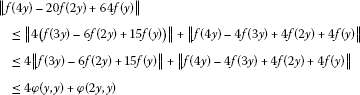(3.3)

for all $y\in X$. Replacing y by x and $g\left(x\right):=f\left(2x\right)-16f\left(x\right)$ in (3.3), we get

$\parallel g\left(x\right)-\frac{1}{4}g\left(2x\right)\parallel \le \phi \left(x,x\right)+\frac{1}{4}\phi \left(2x,x\right)$

for all $x\in X$.

The rest of the proof is similar to the proof of Theorem 2.2. □

Corollary 3.2 Let r be a positive real number with $r<2$, and let $f:X\to Y$ be an even mapping satisfying $f\left(0\right)=0$ and (2.9). Then there exists a unique quadratic mapping ${Q}_{2}:X\to Y$ such that

$\parallel f\left(2x\right)-16f\left(x\right)-{Q}_{2}\left(x\right)\parallel \le \frac{9+{2}^{r}}{4-{2}^{r}}P{\left(x\right)}^{r}$
(3.4)

for all $x\in X$.

Proof Taking $\phi \left(x,y\right)=P{\left(x\right)}^{r}+P{\left(y\right)}^{r}$ for all $x,y\in X$ and choosing $\alpha ={2}^{r-2}$ in Theorem 3.1, we get the desired result. □

Theorem 3.3 Let $\phi :{X}^{2}\to \left[0,\mathrm{\infty }\right)$ be a function such that

$\mathrm{\Phi }\left(x,y\right):=\sum _{j=0}^{\mathrm{\infty }}\frac{1}{{4}^{j}}\phi \left({2}^{j}x,{2}^{j}y\right)<\mathrm{\infty }$

for all $x,y\in X$. Let $f:X\to Y$ be an even mapping satisfying $f\left(0\right)=0$ and (2.2). Then there exists a unique quadratic mapping ${Q}_{2}:X\to Y$ such that

$\parallel f\left(2x\right)-16f\left(x\right)-{Q}_{2}\left(x\right)\parallel \le \mathrm{\Phi }\left(x,x\right)+\frac{1}{4}\mathrm{\Phi }\left(2x,x\right)$

for all $x\in X$.

Proof The proof is similar to the proof of , Theorem 3.2]. □

Remark 3.4 Let $r<2$. Letting $\phi \left(x,y\right)=P{\left(x\right)}^{r}+P{\left(y\right)}^{r}$ for all $x,y\in X$ in Theorem 3.3, we obtain the inequality (3.4). The proof is given in , Theorem 3.2].

Theorem 3.5 Let $\phi :{Y}^{2}\to \left[0,\mathrm{\infty }\right)$ be a function such that there exists an $\alpha <1$ with

$\phi \left(x,y\right)\le \frac{\alpha }{4}\phi \left(2x,2y\right)$

for all $x,y\in Y$. Let $f:Y\to X$ be an even mapping satisfying $f\left(0\right)=0$ and (2.12). Then there exists a unique quadratic mapping ${Q}_{2}:Y\to X$ such that

$P\left(f\left(2x\right)-16f\left(x\right)-{Q}_{2}\left(x\right)\right)\le \frac{\alpha }{1-\alpha }\left(\phi \left(x,x\right)+\frac{1}{4}\phi \left(2x,x\right)\right)$

for all $x\in Y$.

Proof Letting $x=y$ in (2.12), we get

$P\left(f\left(3y\right)-6f\left(2y\right)+15f\left(y\right)\right)\le \phi \left(y,y\right)$
(3.5)

for all $y\in Y$.

Replacing x by 2y in (2.12), we get

$P\left(f\left(4y\right)-4f\left(3y\right)+4f\left(2y\right)+4f\left(y\right)\right)\le \phi \left(2y,y\right)$
(3.6)

for all $y\in Y$.

By (3.5) and (3.6),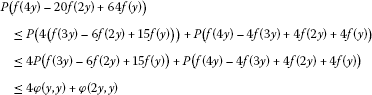(3.7)

for all $y\in Y$. Replacing y by $\frac{x}{2}$ and $g\left(x\right):=f\left(2x\right)-16f\left(x\right)$ in (3.7), we get

$P\left(g\left(x\right)-4g\left(\frac{x}{2}\right)\right)\le 4\phi \left(\frac{x}{2},\frac{x}{2}\right)+\phi \left(x,\frac{x}{2}\right)\le \alpha \left(\phi \left(x,x\right)+\frac{1}{4}\phi \left(2x,x\right)\right)$

for all $x\in Y$.

The rest of the proof is similar to the proof of Theorem 2.6. □

Corollary 3.6 Let r, θ be positive real numbers with $r>2$, and let $f:Y\to X$ be an even mapping satisfying $f\left(0\right)=0$ and (2.19). Then there exists a unique quadratic mapping ${Q}_{2}:Y\to X$ such that

$P\left(f\left(2x\right)-16f\left(x\right)-{Q}_{2}\left(x\right)\right)\le \frac{9+{2}^{r}}{{2}^{r}-4}\theta {\parallel x\parallel }^{r}$
(3.8)

for all $x\in Y$.

Proof Taking $\phi \left(x,y\right)=\theta \left({\parallel x\parallel }^{r}+{\parallel y\parallel }^{r}\right)$ for all $x,y\in X$ and choosing $\alpha ={2}^{2-r}$ in Theorem 3.5, we get the desired result. □

Theorem 3.7 Let $\phi :{Y}^{2}\to \left[0,\mathrm{\infty }\right)$ be a function such that

$\mathrm{\Phi }\left(x,y\right):=\sum _{j=1}^{\mathrm{\infty }}{4}^{j}\phi \left(\frac{x}{{2}^{j}},\frac{y}{{2}^{j}}\right)<\mathrm{\infty }$

for all $x,y\in Y$. Let $f:Y\to X$ be an even mapping satisfying $f\left(0\right)=0$ and (2.12). Then there exists a unique quadratic mapping ${Q}_{2}:Y\to X$ such that

$P\left(f\left(2x\right)-16f\left(x\right)-{Q}_{2}\left(x\right)\right)\le \mathrm{\Phi }\left(x,x\right)+\frac{1}{4}\mathrm{\Phi }\left(2x,x\right)$

for all $x\in Y$.

Proof The proof is similar to the proof of , Theorem 3.1]. □

Remark 3.8 Let $r>2$. Letting $\phi \left(x,y\right)=\theta \left({\parallel x\parallel }^{r}+{\parallel y\parallel }^{r}\right)$ for all $x,y\in X$ in Theorem 3.7, we obtain the inequality (3.8). The proof is given in , Theorem 3.1].

Theorem 3.9 Let $\phi :{X}^{2}\to \left[0,\mathrm{\infty }\right)$ be a function such that there exists an $\alpha <1$ with

$\phi \left(x,y\right)\le 16\alpha \phi \left(\frac{x}{2},\frac{y}{2}\right)$

for all $x,y\in X$. Let $f:X\to Y$ be an even mapping satisfying $f\left(0\right)=0$ and (2.2). Then there exists a unique quartic mapping ${Q}_{4}:X\to Y$ such that

$\parallel f\left(2x\right)-4f\left(x\right)-{Q}_{4}\left(x\right)\parallel \le \frac{1}{1-\alpha }\left(\frac{1}{4}\phi \left(x,x\right)+\frac{1}{16}\phi \left(2x,x\right)\right)$

for all $x\in X$.

Proof Replacing y by x and letting $g\left(x\right):=f\left(2x\right)-4f\left(x\right)$ in (3.3), we get

$\parallel g\left(x\right)-\frac{1}{16}g\left(2x\right)\parallel \le \frac{1}{4}\phi \left(x,x\right)+\frac{1}{16}\phi \left(2x,x\right)$

for all $x\in X$.

The rest of the proof is similar to the proof of Theorem 2.10. □

Corollary 3.10 Let r be a positive real number with $r<4$, and let $f:X\to Y$ be an even mapping satisfying $f\left(0\right)=0$ and (2.9). Then there exists a unique quartic mapping ${Q}_{4}:X\to Y$ such that

$\parallel f\left(2x\right)-4f\left(x\right)-{Q}_{4}\left(x\right)\parallel \le \frac{9+{2}^{r}}{16-{2}^{r}}P{\left(x\right)}^{r}$
(3.9)

for all $x\in X$.

Proof Taking $\phi \left(x,y\right)=P{\left(x\right)}^{r}+P{\left(y\right)}^{r}$ for all $x,y\in X$ and choosing $\alpha ={2}^{r-4}$ in Theorem 3.9, we get the desired result. □

Theorem 3.11 Let $\phi :{X}^{2}\to \left[0,\mathrm{\infty }\right)$ be a function such that

$\mathrm{\Phi }\left(x,y\right):=\sum _{j=0}^{\mathrm{\infty }}\frac{1}{{16}^{j}}\phi \left({2}^{j}x,{2}^{j}y\right)<\mathrm{\infty }$

for all $x,y\in X$. Let $f:X\to Y$ be an even mapping satisfying $f\left(0\right)=0$ and (2.2). Then there exists a unique quartic mapping ${Q}_{4}:X\to Y$ such that

$\parallel f\left(2x\right)-4f\left(x\right)-{Q}_{4}\left(x\right)\parallel \le \frac{1}{4}\mathrm{\Phi }\left(x,x\right)+\frac{1}{16}\mathrm{\Phi }\left(2x,x\right)$

for all $x\in X$.

Proof The proof is similar to the proof of , Theorem 3.4]. □

Remark 3.12 Let $r<4$. Letting $\phi \left(x,y\right)=P{\left(x\right)}^{r}+P{\left(y\right)}^{r}$ for all $x,y\in X$ in Theorem 3.11, we obtain the inequality (3.9). The proof is given in , Theorem 3.4].

Theorem 3.13 Let $\phi :{Y}^{2}\to \left[0,\mathrm{\infty }\right)$ be a function such that there exists an $\alpha <1$ with

$\phi \left(x,y\right)\le \frac{\alpha }{16}\phi \left(2x,2y\right)$

for all $x,y\in Y$. Let $f:Y\to X$ be an even mapping satisfying $f\left(0\right)=0$ and (2.12). Then there exists a unique quartic mapping ${Q}_{4}:Y\to X$ such that

$P\left(f\left(2x\right)-4f\left(x\right)-{Q}_{4}\left(x\right)\right)\le \frac{\alpha }{1-\alpha }\left(\frac{1}{4}\phi \left(x,x\right)+\frac{1}{16}\phi \left(2x,x\right)\right)$

for all $x\in Y$.

Proof Replacing y by $\frac{x}{2}$ and letting $g\left(x\right):=f\left(2x\right)-4f\left(x\right)$ in (3.7), we get

$P\left(g\left(x\right)-16g\left(\frac{x}{2}\right)\right)\le 4\phi \left(\frac{x}{2},\frac{x}{2}\right)+\phi \left(x,\frac{x}{2}\right)\le \alpha \left(\frac{1}{4}\phi \left(x,x\right)+\frac{1}{16}\phi \left(2x,x\right)\right)$

for all $x\in Y$.

The rest of the proof is similar to the proof of Theorem 2.14. □

Corollary 3.14 Let r, θ be positive real numbers with $r>4$, and let $f:Y\to X$ be an even mapping satisfying $f\left(0\right)=0$ and (2.19). Then there exists a unique quartic mapping ${Q}_{4}:Y\to X$ such that

$P\left(f\left(2x\right)-4f\left(x\right)-{Q}_{4}\left(x\right)\right)\le \frac{9+{2}^{r}}{{2}^{r}-16}\theta {\parallel x\parallel }^{r}$
(3.10)

for all $x\in Y$.

Proof Taking $\phi \left(x,y\right)=\theta \left({\parallel x\parallel }^{r}+{\parallel y\parallel }^{r}\right)$ for all $x,y\in X$ and choosing $\alpha ={2}^{4-r}$ in Theorem 3.13, we get the desired result. □

Theorem 3.15 Let $\phi :{Y}^{2}\to \left[0,\mathrm{\infty }\right)$ be a function such that

$\mathrm{\Phi }\left(x,y\right):=\sum _{j=1}^{\mathrm{\infty }}{16}^{j}\phi \left(\frac{x}{{2}^{j}},\frac{y}{{2}^{j}}\right)<\mathrm{\infty }$

for all $x,y\in Y$. Let $f:Y\to X$ be an even mapping satisfying $f\left(0\right)=0$ and (2.12). Then there exists a unique quartic mapping ${Q}_{4}:Y\to X$ such that

$P\left(f\left(2x\right)-4f\left(x\right)-{Q}_{4}\left(x\right)\right)\le \frac{1}{4}\mathrm{\Phi }\left(x,x\right)+\frac{1}{16}\mathrm{\Phi }\left(2x,x\right)$

for all $x\in Y$.

Proof The proof is similar to the proof of , Theorem 3.3]. □

Remark 3.16 Let $r>4$. Letting $\phi \left(x,y\right)=\theta \left({\parallel x\parallel }^{r}+{\parallel y\parallel }^{r}\right)$ for all $x,y\in X$ in Theorem 3.15, we obtain the inequality (3.10). The proof is given in , Theorem 3.3].

We can summarize the corollaries as follows.

Let ${f}_{o}\left(x\right):=\frac{f\left(x\right)-f\left(-x\right)}{2}$ and ${f}_{e}\left(x\right):=\frac{f\left(x\right)+f\left(-x\right)}{2}$. Then ${f}_{o}$ is odd and ${f}_{e}$ is even. ${f}_{o}$, ${f}_{e}$ satisfy the functional equation (1.3). Let ${g}_{o}\left(x\right):={f}_{o}\left(2x\right)-2{f}_{o}\left(x\right)$ and ${h}_{o}\left(x\right):={f}_{o}\left(2x\right)-8{f}_{o}\left(x\right)$. Then ${f}_{o}\left(x\right)=\frac{1}{6}{g}_{o}\left(x\right)-\frac{1}{6}{h}_{o}\left(x\right)$. Let ${g}_{e}\left(x\right):={f}_{e}\left(2x\right)-4{f}_{e}\left(x\right)$ and ${h}_{e}\left(x\right):={f}_{e}\left(2x\right)-16{f}_{e}\left(x\right)$. Then ${f}_{e}\left(x\right)=\frac{1}{12}{g}_{e}\left(x\right)-\frac{1}{12}{h}_{e}\left(x\right)$. Thus

$f\left(x\right)=\frac{1}{6}{g}_{o}\left(x\right)-\frac{1}{6}{h}_{o}\left(x\right)+\frac{1}{12}{g}_{e}\left(x\right)-\frac{1}{12}{h}_{e}\left(x\right).$

Theorem 3.17 Let r be a positive real number with $r<1$. Let $f:X\to Y$ be a mapping satisfying $f\left(0\right)=0$ and (2.9). Then there exist an additive mapping $A:X\to Y$, a quadratic mapping ${Q}_{2}:X\to Y$, a cubic mapping $C:X\to Y$ and a quartic mapping ${Q}_{4}:X\to Y$ such that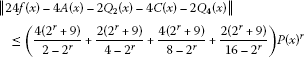for all $x\in X$.

Theorem 3.18 Let r, θ be positive real numbers with $r>4$. Let $f:Y\to X$ be a mapping satisfying $f\left(0\right)=0$ and (2.19). Then there exist an additive mapping $A:Y\to X$, a quadratic mapping ${Q}_{2}:Y\to X$, a cubic mapping $C:Y\to X$ and a quartic mapping ${Q}_{4}:Y\to X$ such that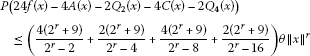for all $x\in Y$.

## 4 Conclusions

Using the fixed point method and direct method, we have proved the Hyers-Ulam stability of an additive-quadratic-cubic-quartic functional equation in paranormed spaces.

## References

1. Fast H: Sur la convergence statistique. Colloq. Math. 1951, 2: 241–244.

2. Steinhaus H: Sur la convergence ordinaire et la convergence asymptotique. Colloq. Math. 1951, 2: 73–74.

3. Fridy JA: On statistical convergence. Analysis 1985, 5: 301–313.

4. Karakus S: Statistical convergence on probabilistic normed spaces. Math. Commun. 2007, 12: 11–23.

5. Mursaleen M: λ -statistical convergence. Math. Slovaca 2000, 50: 111–115.

6. Mursaleen M, Mohiuddine SA: On lacunary statistical convergence with respect to the intuitionistic fuzzy normed space. J. Comput. Appl. Math. 2009, 233: 142–149. 10.1016/j.cam.2009.07.005

7. Šalát T: On the statistically convergent sequences of real numbers. Math. Slovaca 1980, 30: 139–150.

8. Kolk E: The statistical convergence in Banach spaces. Tartu Ülik. Toim. 1991, 928: 41–52.

9. Wilansky A: Modern Methods in Topological Vector Space. McGraw-Hill, New York; 1978.

10. Ulam SM: A Collection of the Mathematical Problems. Interscience, New York; 1960.

11. Hyers DH: On the stability of the linear functional equation. Proc. Natl. Acad. Sci. USA 1941, 27: 222–224. 10.1073/pnas.27.4.222

12. Aoki T: On the stability of the linear transformation in Banach spaces. J. Math. Soc. Jpn. 1950, 2: 64–66. 10.2969/jmsj/00210064

13. Rassias TM: On the stability of the linear mapping in Banach spaces. Proc. Am. Math. Soc. 1978, 72: 297–300. 10.1090/S0002-9939-1978-0507327-1

14. Gǎvruta P: A generalization of the Hyers-Ulam-Rassias stability of approximately additive mappings. J. Math. Anal. Appl. 1994, 184: 431–436. 10.1006/jmaa.1994.1211

15. Rassias, TM: Problem 16; 2. Report of the 27th International Symp. on Functional Equations. Aequ. Math. 39, 292–293 (1990)

16. Gajda Z: On stability of additive mappings. Int. J. Math. Math. Sci. 1991, 14: 431–434. 10.1155/S016117129100056X

17. Rassias TM, Šemrl P: On the behaviour of mappings which do not satisfy Hyers-Ulam stability. Proc. Am. Math. Soc. 1992, 114: 989–993. 10.1090/S0002-9939-1992-1059634-1

18. Czerwik P: Functional Equations and Inequalities in Several Variables. World Scientific, Singapore; 2002.

19. Hyers DH, Isac G, Rassias TM: Stability of Functional Equations in Several Variables. Birkhäuser, Basel; 1998.

20. Skof F: Proprietà locali e approssimazione di operatori. Rend. Semin. Mat. Fis. Milano 1983, 53: 113–129. 10.1007/BF02924890

21. Cholewa PW: Remarks on the stability of functional equations. Aequ. Math. 1984, 27: 76–86. 10.1007/BF02192660

22. Czerwik S: On the stability of the quadratic mapping in normed spaces. Abh. Math. Semin. Univ. Hamb. 1992, 62: 59–64. 10.1007/BF02941618

23. Jun K, Lee Y: A generalization of the Hyers-Ulam-Rassias stability of the Pexiderized quadratic equations. J. Math. Anal. Appl. 2004, 297: 70–86. 10.1016/j.jmaa.2004.04.009

24. Jung S: Hyers-Ulam-Rassias Stability of Functional Equations in Mathematical Analysis. Hadronic Press, Palm Harbor; 2001.

25. Rassias TM: Functional Equations and Inequalities. Kluwer Academic, Dordrecht; 2000.

26. Rassias TM: On the stability of functional equations in Banach spaces. J. Math. Anal. Appl. 2000, 251: 264–284. 10.1006/jmaa.2000.7046

27. Rassias TM: On the stability of functional equations and a problem of Ulam. Acta Appl. Math. 2000, 62: 23–130. 10.1023/A:1006499223572

28. Czerwik S: Stability of Functional Equations of Ulam-Hyers-Rassias Type. Hadronic Press, Palm Harbor; 2003.

29. Rassias TM: Functional Equations, Inequalities and Applications. Kluwer Academic, Dordrecht; 2003.

30. Jun K, Kim H: The generalized Hyers-Ulam-Rassias stability of a cubic functional equation. J. Math. Anal. Appl. 2002, 274: 867–878. 10.1016/S0022-247X(02)00415-8

31. Lee S, Im S, Hwang I: Quartic functional equations. J. Math. Anal. Appl. 2005, 307: 387–394. 10.1016/j.jmaa.2004.12.062

32. Gordji ME, Kaboli-Gharetapeh S, Park C, Zolfaghari S: Stability of an additive-cubic-quartic functional equation. Adv. Differ. Equ. 2009., 2009: Article ID 395693

33. Gordji ME, Abbaszadeh S, Park C: On the stability of a generalized quadratic and quartic type functional equation in quasi-Banach spaces. J. Inequal. Appl. 2009., 2009: Article ID 153084

34. Diaz J, Margolis B: A fixed point theorem of the alternative for contractions on a generalized complete metric space. Bull. Am. Math. Soc. 1968, 74: 305–309. 10.1090/S0002-9904-1968-11933-0

35. Cădariu L, Radu V: Fixed points and the stability of Jensen’s functional equation. J. Inequal. Pure Appl. Math. 2003., 4(1): Article ID 4

36. Isac G, Rassias TM: Stability of ψ -additive mappings: applications to nonlinear analysis. Int. J. Math. Math. Sci. 1996, 19: 219–228. 10.1155/S0161171296000324

37. Cădariu L, Radu V: On the stability of the Cauchy functional equation: a fixed point approach. Grazer Math. Ber. 2004, 346: 43–52.

38. Cădariu L, Radu V: Fixed point methods for the generalized stability of functional equations in a single variable. Fixed Point Theory Appl. 2008., 2008: Article ID 749392

39. Jung Y, Chang I: The stability of a cubic type functional equation with the fixed point alternative. J. Math. Anal. Appl. 2005, 306: 752–760. 10.1016/j.jmaa.2004.10.017

40. Mirzavaziri M, Moslehian MS: A fixed point approach to stability of a quadratic equation. Bull. Braz. Math. Soc. 2006, 37: 361–376. 10.1007/s00574-006-0016-z

41. Park C: Fixed points and Hyers-Ulam-Rassias stability of Cauchy-Jensen functional equations in Banach algebras. Fixed Point Theory Appl. 2007., 2007: Article ID 50175

42. Park C: Generalized Hyers-Ulam-Rassias stability of quadratic functional equations: a fixed point approach. Fixed Point Theory Appl. 2008., 2008: Article ID 493751

43. Radu V: The fixed point alternative and the stability of functional equations. Fixed Point Theory 2003, 4: 91–96.

44. Miheţ D, Radu V: On the stability of the additive Cauchy functional equation in random normed spaces. J. Math. Anal. Appl. 2008, 343: 567–572. 10.1016/j.jmaa.2008.01.100

45. Park, C: An AQCQ-functional equation in paranormed spaces. Preprint

## Author information

Authors

### Corresponding author

Correspondence to Choonkil Park.

### Competing interests

The author declares that they have no competing interests.

## Rights and permissions

Reprints and Permissions

Park, C. Stability of an AQCQ-functional equation in paranormed spaces. Adv Differ Equ 2012, 148 (2012). https://doi.org/10.1186/1687-1847-2012-148

• Accepted:

• Published:

• DOI: https://doi.org/10.1186/1687-1847-2012-148

### Keywords

• Hyers-Ulam stability
• paranormed space
• fixed point